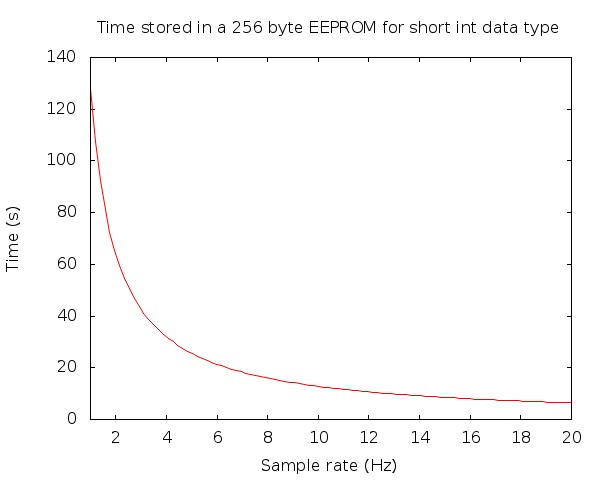# Telemetry backup, EEPROM

Sending data over radio frequency is subject to connectivity flaws; thus it is important to have some data backup mechanism. The most sensible option would be to attach an SD module to the μC because that would allow for tons of parameters to be stored. But that poses a weight penalty, though negligible.

As we just want to save a few seconds of altitude recordings, the built-in EEPROM memory will suffice. Essentially, an EEPROM is a type of non-volatile used in computers and other electronic devices to store small amounts of data that must be saved when power is removed. A very small amount of data, because the PIC18LF13K50 has a data EEPROM memory range of only 256 bytes. Those 256 bytes of data storage capacity will grant us a few seconds of data backup; time which can be estimated using the following equation,$\displaystyle \text{time recorded} = \frac{256\,\mathrm{bytes}}{n\,\mathrm{bytes/sample}\cdot \text{sample rate in Hz}}$

If we recall, the BMP085 barometric pressure sensor generates temperature and pressure readings in the long (32 bit) format. Storing those raw values would take away a lot of memory space; so, a better approach would be to process those readings and store the computed altitude estimate. Altitude can be assigned to a short integer data type (16 bit) retaining one decimal of accuracy, i.e., measuring an altitude range from 0.0 to 6553.5 m. Of course, integer data types don’t know anything about floating points, but I know that I will be storing values in decimeters, so that’ll do the trick. And considering that the barometric pressure sensor has a sensibility of about 0.3 m, decimeters will do fine.Figure 1. Time of flight stored in EEPROM vs sample rate.

Now, how to access the EEPROM memory? Although it is not directly mapped in either the register file of program memory space, it is inderectly addressed through the Special Function Registers (SFRs). The EEPROM is otherwise readable and writable during normal operation using four SFRs. They are:

When interfacing to the data memory block, EEDATA holds the 8-bit data for read/write and the EEADR:EEADRH register pair hold the address of the EEPROM location being accessed. On the other hand, access to the data EEPROM is controlled by two registers, EECON1 and EECON2.

EECON1: The  control register for data and program memory access,

• EEPGD: Flash Program (1) or Data EEPROM Memory (0) Select bit
• CFGS: Configuration (1) of Flash Program/Data EEPROM Memory (0) Select bit
• FREE: Flash Row (Block) Erase Enable (1) bit
• WRERR: Flash Program/Data EEPROM Error Flag bit
• WREN: Flash Program/Data EEPROM Write Enable (1) bit
• WR: Write Control bit
• RD: Read Control bit

An snippet of working code is shown below.

[code lang=”c”]
#include <p18LF14K50>

void High_Int_Handler (void);

unsigned char ee_dir, i;
unsigned char value;

#pragma code High_Int = 0x08
void High_Int(void) {
_asm goto High_Int_Handler _endasm
}
//#pragma code

#pragma interrupt High_Int_Handler

void High_Int_Handler (void) { // High priority interrupts
if (INTCONbits.TMR0IF) {
INTCONbits.TMR0IF = 0; // Restart TMR0
TMR0H = 15536/256; //
TMR0L = 15536%256; //

/* Gather sensor values and process them */

value = msb; // MSB for 16 bit altitude value
value = lsb; // LSB for 16 bit altitude value
for (i = 0; i < 2; i++) {
EEADR = ee_dir + i; // Data Memory Address to write
EEDATA = value[i]; // Data Memory value to write
EECON1bits.WREN = 1; // Data EEPROM Write Enable
INTCONbits.GIE = 0; // Disable Interrupts
EECON2 = 0x55; // Required sequence
EECON2 = 0xAA; // Required sequence
EECON1bits.WR = 1; // Set WR bit to begin write
INTCONbits.GIE = 1; // Enable Interrupts
while (EECON1bits.WR == 1); // On write complete,
EECON1bits.WREN = 0; // disable writes
}

ee_dir = ee_dir + 2; // Increase starting Memory position by two
if (ee_dir == 256) { // If memory limit reached,
T0CONbits.TMR0ON=0; // cease sampling
}
}
}

void main (void) {
EECON1=0x00; // Access EEPROM (EEPGD=’0′ and CFGS=’0′)
ee_dir=0;

while (1);
}
[/code]

Finally, in ISIS Proteus it is possible to see what’s written into the Data EEPROM Memory just enabling the option in the Debug tab as shown in Figure 2.